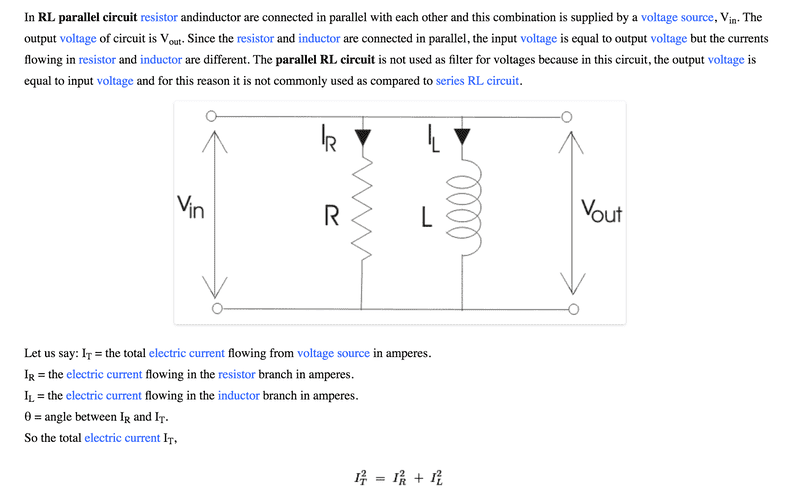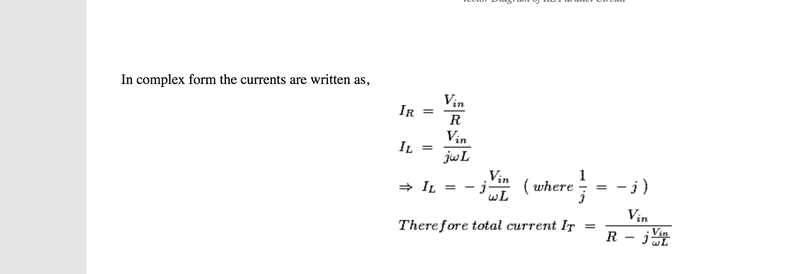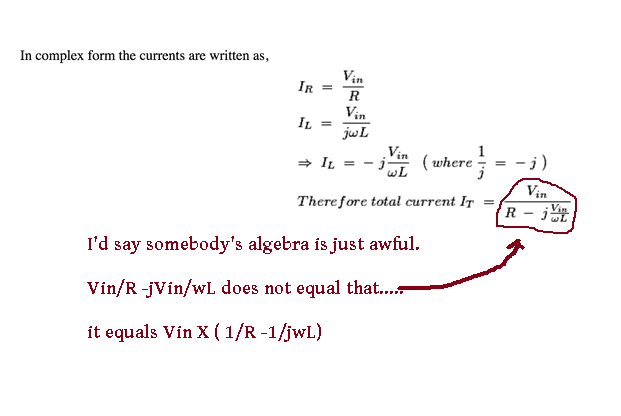# RL Circuit Refresher

• jendrix

#### jendrix

Hi, I have just been refreshing my theory and was going over a parallel RL circuit, like this one.I tried some values as a test but it doesn't give me the correct answer, for total current. eg if I set
V=10V
R=2.5ohm
jXl=5ohm

Then IR=10/2.5 =4A

IL=10/5=2A

IT=√42 +22 =4.47A

However when I try the formula from the second answer I get 10/(2.5-2j) which following the maths gives me 2.44+1.95j=3.12A

Am I going wrong somewhere or is it the formula?

Thanks

#### Attachments

However when I try the formula from the second answer I get 10/(2.5-2j) which following the maths gives me 2.44+1.95j=3.12AA 'Site', you said ? If it's on the internet it must be right, eh ? See my signature.....

see if that works any better.

old jim

#### Attachments

•jendrix and Delta2
Oops ----- make that it equals Vin X ( 1/R -j1/ωL )

i put my j in wrong place .•Delta2
Oops ----- make that it equals Vin X ( 1/R -j1/ωL )

i put my j in wrong place .Seems we can't even trust Old Jim when he posts at the internet with a computer, cause we can never trust a computer with anything importantOk seriously speaking that was a minor mistake, I didn't notice it either, I just noticed the huge mistake in the algebra in the OP.

•jim hardy
i do that all the time. I'm just clumsy.

Thanks @Delta2

•jendrix
i do that all the time. I'm just clumsy.

Thanks @Delta2

Thanks Jim for clearing that up

•jim hardy and Delta2